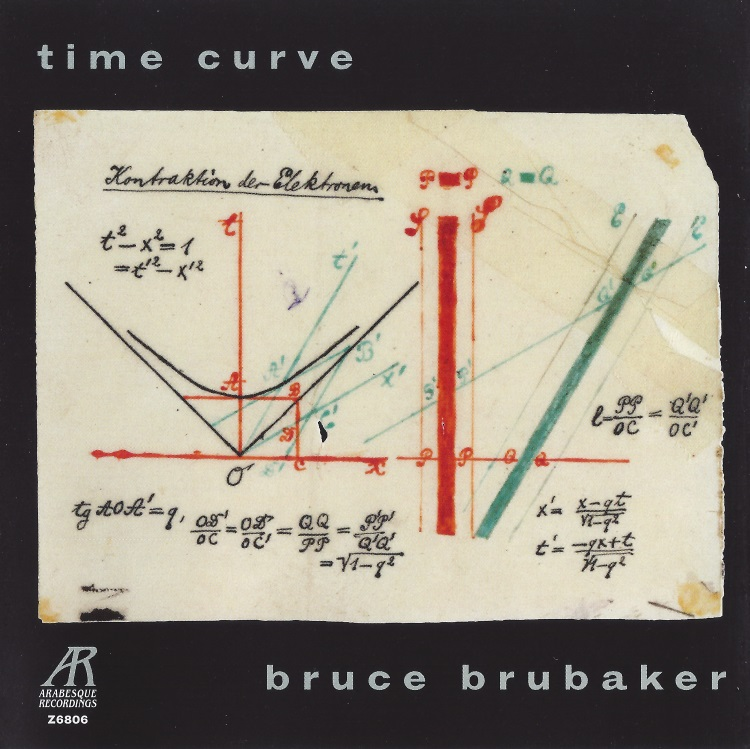AVAILABLE AT

# Z6806

1 Six Etudes for Piano (q = 168)
2 Six Etudes for Piano (q = 108)
3 Six Etudes for Piano (q = 132)
4 Six Etudes for Piano (q = 104)
5 Six Etudes for Piano (q = 96)
6 Six Etudes for Piano (q = 176)
7 The Time Curve Preludes, Book I (q = 104)
8 The Time Curve Preludes, Book I: II (q = 104)
9 The Time Curve Preludes, Book I: III (q = 126)
10 The Time Curve Preludes, Book I: IV (q = 132)
11 The Time Curve Preludes, Book I (q = 132)
12 The Time Curve Preludes, Book I: VI (q = 104)
13 The Time Curve Preludes, Book I: VII (q = 72)
14 The Time Curve Preludes, Book I: VIII (q = 76)
15 The Time Curve Preludes, Book I: IX (q = 96)
16 The Time Curve Preludes, Book I (q = 192)
17 The Time Curve Preludes, Book I: XI (q = 80 – Freely, with great rubato)
18 The Time Curve Preludes, Book I: XII (q = 124)